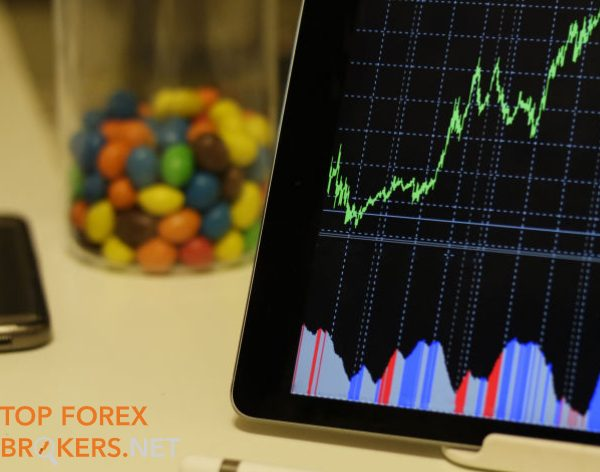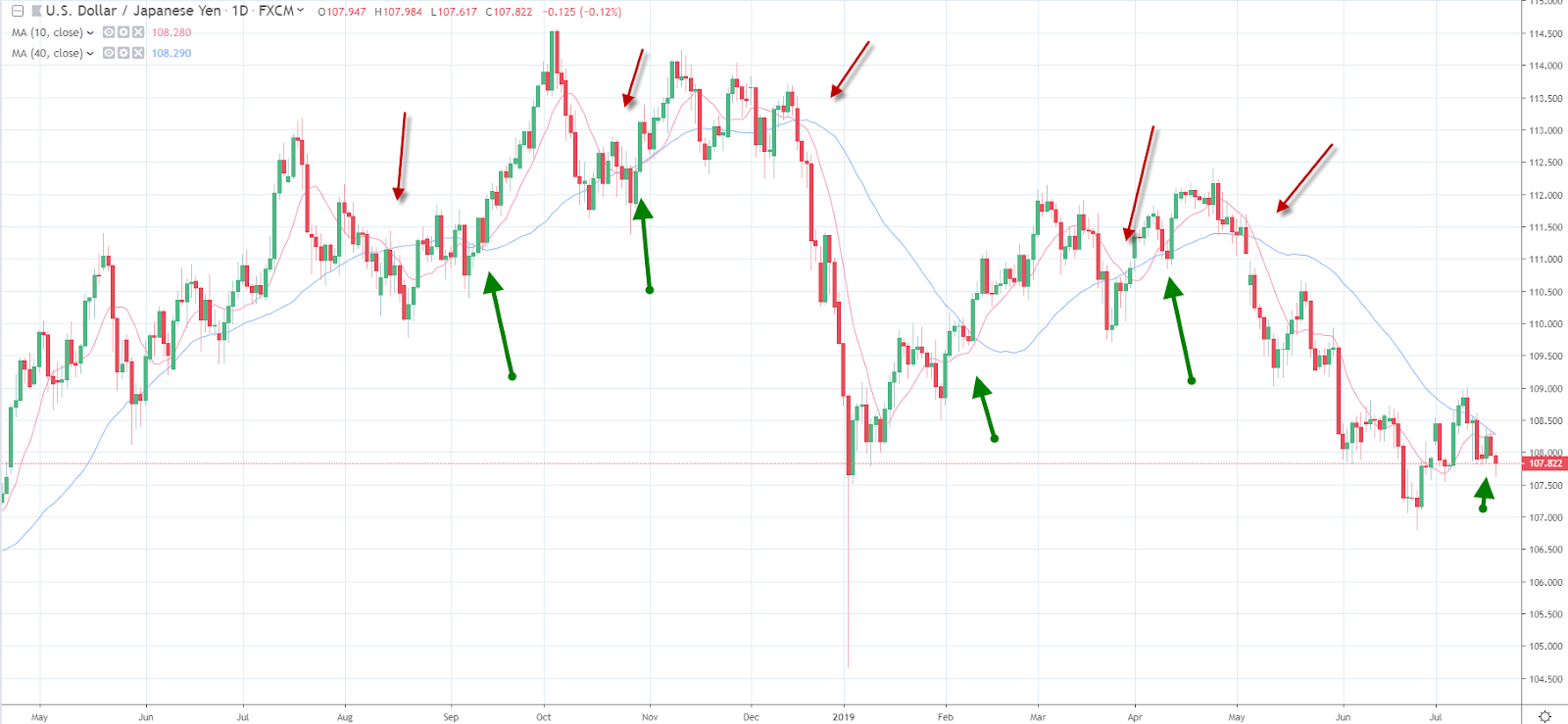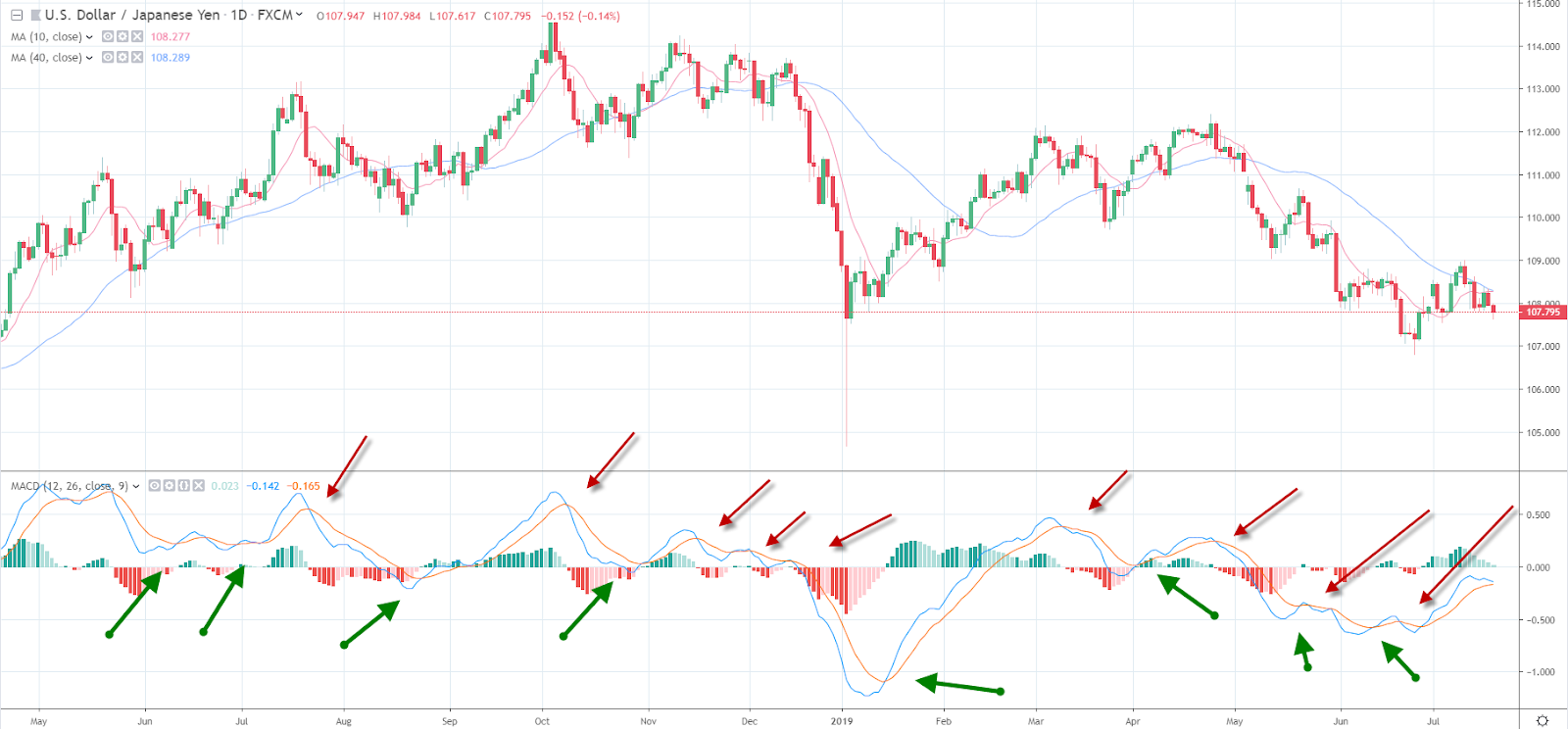by Greyson on July 23, 2019

# Three Basic Trading Strategies for BeginnersThere are many ways to trade the forex markets. Prior to risking your capital, you need to determine the type of trading strategy you plan to use to take advantage of the marketYour strategies could be fundamental where you look for changes in the macro-environment to take a view on a currency pair, or you could use technical analysis. This is the study of past price action which helps you predict the future movements of a currency pair. Here are three basic trading strategies that a beginning trader can use.

## Trend Following

A trend is the movement of price in a specific direction that continues to perpetuate. The markets in theory only trend 30% of the time, which means that it’s important to jump aboard as soon as a trend starts. One of the easiest trend-following strategies to follow is the moving average crossover strategy. A simple moving average is the average of a specific number of days. For example, a ten-day moving average takes the simple average of the past 10-days. On day eleven, the first day in the averaging period is dropped from the calculation. A moving average helps remove some of the choppiness of day to day changes in an exchange rate.

## A Simple Moving Average Crossover Strategy

simple moving average crossover strategy tells you to buy an exchange rate when a slower moving average (such as the 10-day moving average) crosses above a longer-term moving average (such as the 40-day moving average). You can see from the chart of the USD/JPY there are several signals that tell you when to purchase the USD/JPY currency pair and when to sell the USD/JPY currency pair.The risk management of this strategy can be as simple as reversing your position when the reverse signal appears.

## Exponential Moving Average Crossover

Another similar trading strategy is the exponential moving average crossover strategy. This trading strategy increases the relevance of the recent days, by weighting the current period more than the prior period of the moving average. Since a moving average is a lagging indicator, the exponential moving average attempt to generate a trend signal ahead of a simple moving average crossover trading strategy.

A simple momentum trading strategy is the Moving Average Convergence Divergence Crossover Strategy. The MACD generates a momentum buy and signal.The MACD evaluated momentum by analyzing the difference between a short term moving average (the 12-day moving average) and a longer-term moving average (the 26-day moving average). This is called the MACD line. It then compares this difference (the MACD line) to a moving average of the difference (the MACD signal line). When the MACD line crosses over the MACD signal line a buy signal is generated (green arrows). When the MACD line cross below the MACD signal line (the red arrows), a sell signal is generated. The risk management of this strategy can be as simple as exiting a position when the reverse signal occurs.

### By Greyson